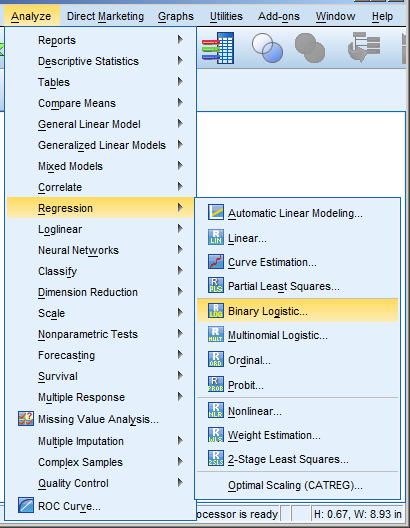# plum

### SPSS Procedures for Logistic Regression

May 15th, 2014 by

Need to run a logistic regression in SPSS? Turns out, SPSS has a number of procedures for running different types of logistic regression.

Some types of logistic regression can be run in more than one procedure.  For some unknown reason, some procedures produce output others don’t.  So it’s helpful to be able to use more than one.

### Logistic RegressionLogistic Regression can be used only for binary dependent (more…)